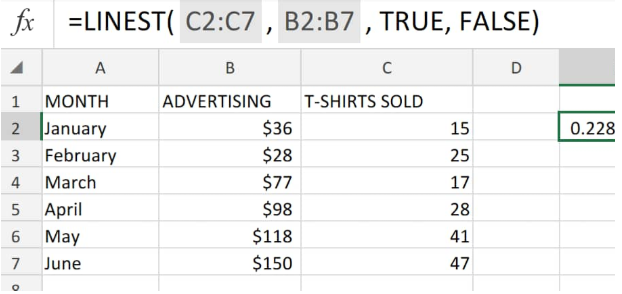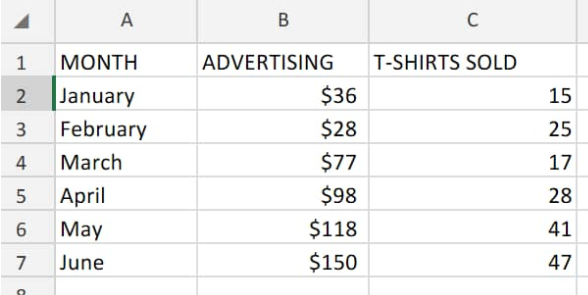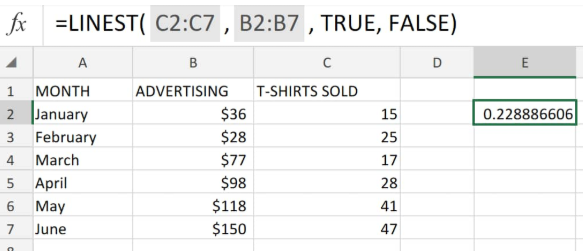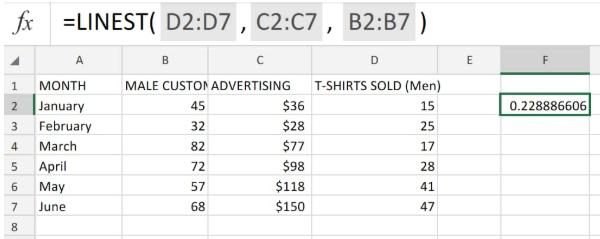Get instant live expert help with Excel or Google Sheets“My Excelchat expert helped me in less than 20 minutes, saving me what would have been 5 hours of work!”

#### Post your problem and you'll get expert help in seconds

Your message must be at least 40 characters
Our professional experts are available now. Your privacy is guaranteed.

# How to use Linest Excel

This article explains how to use the LINEST function with a description of its formula syntax and examples.

Linest is an in-built mathematical function of excel.

It is designed to carry out linear regression analysis and return a set of related statistics.Figure 1. of Linest Function in Excel

## Formula Syntax

`=LINEST( y_values, x_values, constant)`

Y_values are a specific array of independent values within our linear equation.

X_values (optional). A known array of dependent values within our linear equation. In cases where this parameter isn’t specified, our assumed dependent values should be 1,2,3,4,… with the same amount of values as our y_values.

Constant (optional) this can be TRUE or FALSE. Once this parameter is not included, the LINEST function will assume it to be TRUE.

## How to use the LINEST function

We will demonstrate the theory and calculations of using Linest with the following simple steps;

The LINEST function determines the statistical Linest values for data arranged in a straight line explaining the relationship between an independent variable and a dependent variable(s) and returns a numerical value which best describes our data.

Let’s say we have the monthly advertising and sales records at a clothing store for six months.

1. We begin by entering our corresponding data values for each month into separate columns of our excel worksheet.Figure 2. of Linest Function

1. The Linest formula we shall enter into cell D2 of our worksheet example is as follows:

`=LINEST(C2:C7, B2:B7),TRUE,FALSE)`Figure 3. of Linest Function in Excel

If found to be TRUE, the LINEST output will be a number value having additional regression statistics.

If omitted, or found to be FALSE, LINEST function returns error.

LINEST can also be combined with other functions for use in calculating different types of model statistics which are linear with unknown parameters, such as polynomials, logarithmic, exponentials, and also power series.

Since the Linest function calculates a set of values, it should be entered into our worksheet in an array format.

This might make the LINEST function a bit tricky to use because we should not only input our formula syntax correctly, but also interpret our Linest output properly.

## Additional Statistics for Linest Formula

To obtain even more statistical data for our linear analysis, we can enter another TRUE operation after the last argument in our Linest array.

Applied to our worksheet example, the Linest formula syntax appears in the following shape:Figure 4. of Linest Function in Excel

## Instant Connection to an Excel Expert

Most of the time, the problem you will need to solve will be more complex than a simple application of a formula or function. If you want to save hours of research and frustration, try our live Excelchat service! Our Excel Experts are available 24/7 to answer any Excel question you may have. We guarantee a connection within 30 seconds and a customized solution within 20 minutes.